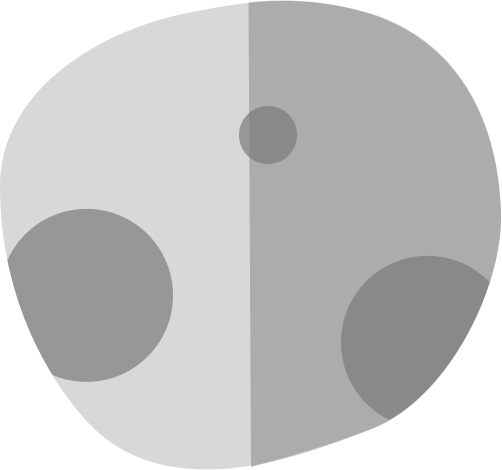# Designing PlanetsYou are asked to design a planet of mass $M$ and uniform density $\rho$ such that it has the maximum possible acceleration due to gravity $g$ at a point on its surface.

Find the shape of this planet, and then find $g.$

Note: $G$ is the universal gravitational constant.

×

Problem Loading...

Note Loading...

Set Loading...